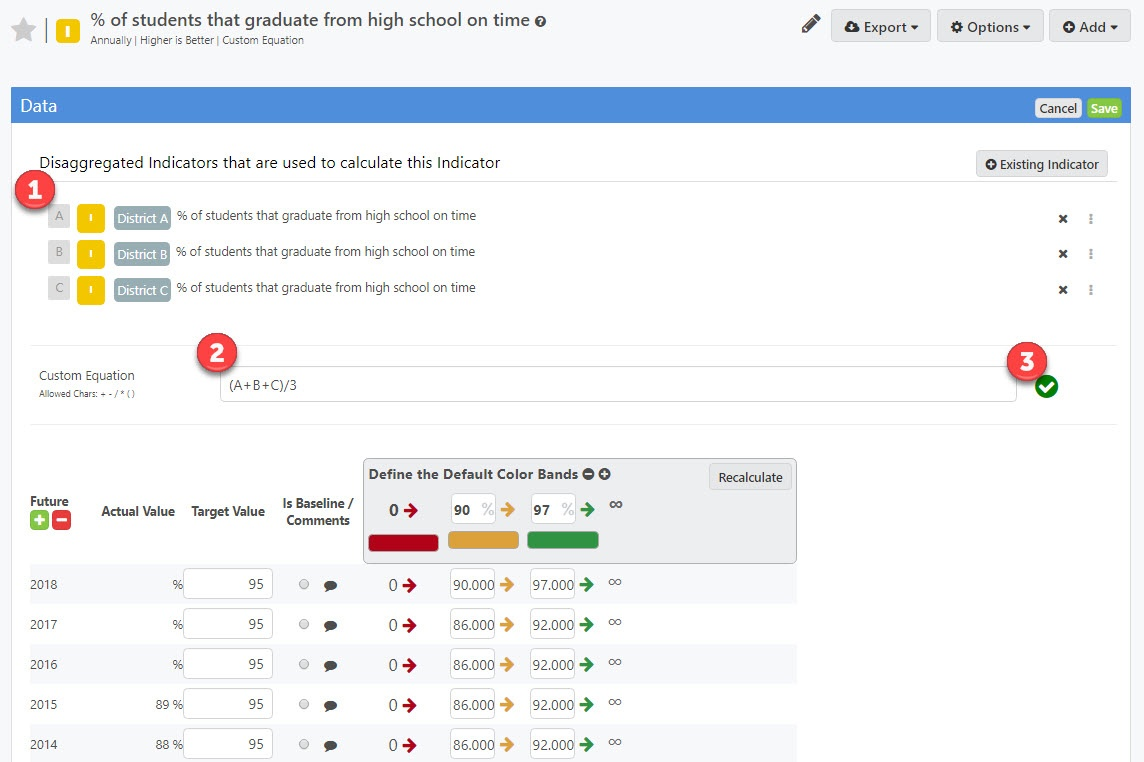# Calculated Measures - Custom Equations

## The custom equation builder allows you to create your own equations for combining data values from other measures

The Custom calculation type is a powerful tool that allows you to write your own free-form equations.

This can be used for getting values like attendance or graduation rates from population numbers. It is similar to writing an equation in Microsoft Excel except that in Clear Impact Scorecard it can dynamically run the calculation across all time periods where data is available in the disaggregated measures.

To use the custom calculation set up a measure with the calculation type of "Custom Calculation" using the steps on the Calculated Measures article here.

Once you set up your measure you can add disaggregated measures on the Data tab. When you add measures, you will notice a letter off to the left side of the measure name. This letter is the reference code you can use to write your equation.1. The measure's reference letter for use in the custom equation box
2. The custom equation entry box. Enter your equation here using the allowed characters of letters, numbers, and + - / * ( )
3. The validation feedback icon. This icon will switch between a green check mark and red exclamation mark to let you know if your equation is valid or not.

Once you hit Save the software will loop through each time period and run the equation on any time periods where data values exist for all the disaggregated measures.